Last week I blogged about the broken-stick problem in probability, which reminded me that the broken-stick model is one of the many techniques that have been proposed for choosing the number of principal components to retain during a principal component analysis. Recall that for a principal component analysis (PCA) of p variables, a goal is to represent most of the variation in the data by using k new variables, where hopefully k is much smaller than p. Thus PCA is known as a dimension-reduction algorithm.

Many researchers have proposed methods for choosing the number of principal components. Some methods are heuristic, others are statistical. No method is perfect. Often different techniques result in different suggestions.

This article uses SAS to implement the broken stick model and compares that method with three other simple rules for dimension reduction. A few references are provided at the end of this article.

### A principal component analysis by using PROC PRINCOMP

Let's start with an example. In SAS, you can use the PRINCOMP procedure to conduct a principal component analysis. The following example is taken from the Getting Started example in the PROC PRINCOMP documentation. The program analyzes seven crime rates for the 50 US states in 1977. (The documentation shows how to generate the data set.) The following call generates a scree plot, which shows the proportion of variance explained by each component. It also writes the Eigenvalues table to a SAS data set:

```proc princomp data=Crime plots=scree; var Murder Rape Robbery Assault Burglary Larceny Auto_Theft; id State; ods output Eigenvalues=Evals; run;```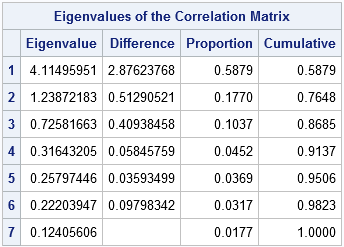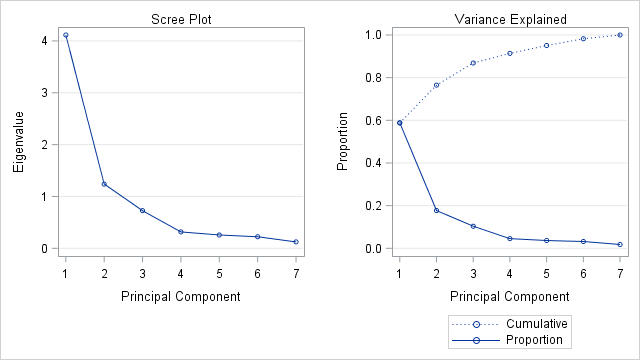The panel shows two graphs that plot the numbers in the "Eigenvalues of the Correlation Matrix" table. The plot on the left is the scree plot, which is a graph of the eigenvalues. The sum of the eigenvalues is 7, which is the number of variables in the analysis. If you divide each eigenvalue by 7, you obtain the proportion of variance that each principal component explains. The graph on the right plots the proportions and the cumulative proportions.

### The scree plot as a guide to retaining components

The scree plot is my favorite graphical method for deciding how many principal components to keep. If the scree plot contains an "elbow" (a sharp change in the slopes of adjacent line segments), that location might indicate a good number of principal components (PCs) to retain. For this example, the scree plot shows a large change in slopes at the second eigenvalue and a smaller change at the fourth eigenvalue. From the graph of the cumulative proportions, you can see that the first two PCs explain 76% of the variance in the data, whereas the first four PCs explain 91%.

If "detect the elbow" is too imprecise for you, a more precise algorithm is to start at the right-hand side of the scree plot and look at the points that lie (approximately) on a straight line. The leftmost point along the trend line indicates the number of components to retain. (In geology, "scree" is rubble at the base of a cliff; the markers along the linear trend represent the rubble that can be discarded.) For the example data, the markers for components 4–7 are linear, so components 1–4 would be kept. This rule (and the scree plot) was proposed by Cattell (1966) and revised by Cattell and Jaspers (1967).

### How does the broken-stick model choose components?

D. A. Jackson (1993) says that the broken-stick method is one of the better methods for choosing the number of PCs. The method provides "a good combination of simplicity of calculation and accurate evaluation of dimensionality relative to the other statistical approaches" (p. 2212). The broken-stick model retains components that explain more variance than would be expected by randomly dividing the variance into p parts. As I discussed last week, if you randomly divide a quantity into p parts, the expected proportion of the kth largest piece is (1/p)Σ(1/i) where the summation is over the values i=k..p. For example, if p=7 then
E1 = (1 + 1/2 + 1/3 + 1/4 + 1/5 + 1/6 + 1/7) / 7 = 0.37,
E2 = (1/2 + 1/3 + 1/4 + 1/5 + 1/6 + 1/7) / 7 = 0.228,
E3 = (1/3 + 1/4 + 1/5 + 1/6 + 1/7) / 7 = 0.156, and so forth.

I think of the "expected proportions" as corresponding to a null model that contains uncorrelated (noise) variables. If you plot the eigenvalues of the correlation matrix against the broken-stick proportions, the observed proportions that are higher than the expected proportions indicate of how many principal component to keep.

### The broken-stick model for retaining components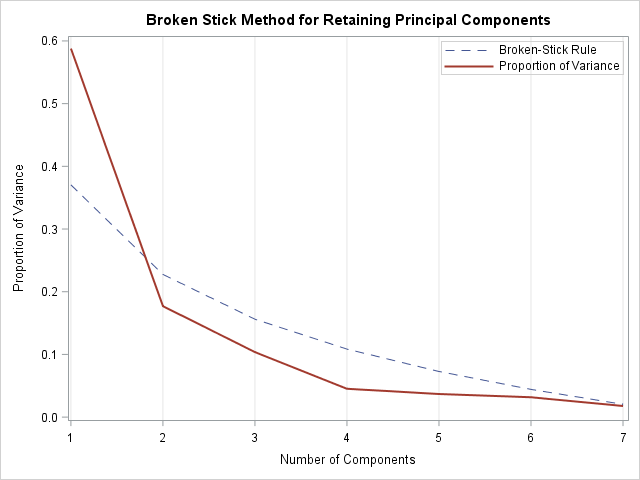The plot to the right shows the scree plot overlaid on a dashed curve that indicates the expected proportions that result from a broken-stick model. An application of the broken-stick model keeps one PC because only the first observed proportion of variance is higher than the corresponding broken-stick proportion.

How can you compute the points on the dashed curve? The expected proportions in the broken-stick model for p variables are proportional to the cumulative sums of the sequence of ratios {1/p, 1/(p-1), ..., 1}. You can use the CUSUM function in SAS/IML to compute a cumulative sum of a sequence, as shown below. Notice that the previous call to PROC PRINCOMP used the ODS OUTPUT statement to create a SAS data set that contains the values in the Eigenvalue table. The SAS/IML program reads in that data and compares the expected proportions to the observed proportions. The number of components to retain is computed as the largest integer k for which the first k components each explain more variance than the broken-stick model (null model).

```proc iml; use Evals; read all var {"Number" "Proportion"}; close;   /* Broken Stick (Joliffe 1986; J. E. Jackson, p. 47) */ /* For random p-1 points in [0,1], expected lengths of the p subintervals are: */ p = nrow(Proportion); g = cusum(1 / T(p:1)) / p; /* expected lengths of intervals (smallest to largest) */ ExpectedLen = g[p:1]; /* reverse order: largest to smallest */   keep = 0; /* find first k for which ExpectedLen[i] < Proportion[i] if i<=k */ do i = 1 to p while(ExpectedLen[i] < Proportion[i]); keep = i; end; print Proportion ExpectedLen keep;```As seen in the graph, only the first component is retained under the broken-stick model.

### Average of eigenvalues

The average-eigenvalue test (Kaiser-Guttman test) retains the eigenvalues that exceed the average eigenvalue. For a p x p correlation matrix, the sum of the eigenvalues is p, so the average value of the eigenvalues is 1. To account for sampling variability, Jolliffe (1972) suggested a more liberal criterion: retain eigenvalues greater than 0.7 times the average eigenvalue. These two suggestions are implemented below:

```/* Average Root (Kaiser 1960; Guttman 1954; J. E. Jackson, p. 47) */ mean = mean(Proportion); keepAvg = loc( Proportion >= mean )[<>];   /* Scaled Average Root (Joliffe 1972; J. E. Jackson, p. 47-48) */ keepScaled = loc( Proportion >= 0.7*mean )[<>]; print keepAvg keepScaled;```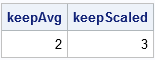### Create the broken-stick graph

For completeness, the following statement write the broken-stick proportions to a SAS data set and call PROC SGPLOT to overlay the proportion of variance for the observed data on the broken-stick model:

```/* write expected proportions for broken-stick model */ create S var {"Number" "Proportion" "ExpectedLen"}; append; close; quit;   title "Broken Stick Method for Retaining Principal Components"; proc sgplot data=S; label ExpectedLen = "Broken-Stick Rule" Proportion = "Proportion of Variance" Number = "Number of Components"; series x=Number y=ExpectedLen / lineattrs=(pattern=dash); series x=Number y=Proportion / lineattrs=(thickness=2); keylegend / location=inside position=topright across=1; xaxis grid; yaxis label = "Proportion of Variance"; run;```

### Summary

Sometimes people ask why PROC PRINCOMP doesn't automatically choose the "correct" number of PCs to use for dimension reduction. This article describes four popular heuristic rules, all which give different answers! The rules in this article are the scree test (2 or 4 components), the broken-stick rule (1 component), the average eigenvalue rule (2 components), and the scaled eigenvalue rule (3 components).

So how should a practicing statistician decide how many PCs to retain? First, remember that these guidelines do not tell you how many components to keep, they merely make suggestions. Second, recognize that any reduction of dimension requires a trade-off between accuracy (high dimensions) and interpretability (low dimensions). Third, these rules—although helpful—cannot replace domain-specific knowledge of the data. Try each suggestion and see if the resulting model contains the features in the data that are important for your analysis.

The post Dimension reduction: Guidelines for retaining principal components appeared first on The DO Loop.Skewness is a measure of the asymmetry of a univariate distribution. I have previously shown how to compute the skewness for data distributions in SAS. The previous article computes Pearson's definition of skewness, which is based on the standardized third central moment of the data.

Moment-based statistics are sensitive to extreme outliers. A single extreme observation can radically change the mean, standard deviation, and skewness of data. It is not surprising, therefore, that there are alternative definitions of skewness. One robust definition of skewness that is intuitive and easy to compute is a quantile definition, which is also known as the Bowley skewness or Galton skewness.

### A quantile definition of skewness

The quantile definition of skewness uses Q1 (the lower quartile value), Q2 (the median value), and Q3 (the upper quartile value). You can measure skewness as the difference between the lengths of the upper quartile (Q3-Q2) and the lower quartile (Q2-Q1), normalized by the length of the interquartile range (Q3-Q1). In symbols, the quantile skewness γQ isYou can visualize this definition by using the figure to the right.For a symmetric distribution, the quantile skewness is 0 because the length Q3-Q2 is equal to the length Q2-Q1. If the right length (Q3-Q2) is larger than the left length (Q2-Q1), then the quantile skewness is positive. If the left length is larger, then the quantile skewness is negative. For the extreme cases when Q1=Q2 or Q2=Q3, the quantile skewness is ±1. Consequently, whereas the Pearson skewness can be any real value, the quantile skewness is bounded in the interval [-1, 1]. The quantile skewness is not defined if Q1=Q3, just as the Pearson skewness is not defined when the variance of the data is 0.

There is an intuitive interpretation for the quantile skewness formula. Recall that the relative difference between two quantities R and L can be defined as their difference divided by their average value. In symbols, RelDiff = (R - L) / ((R+L)/2). If you choose R to be the length Q3-Q2 and L to be the length Q2-Q1, then quantile skewness is half the relative difference between the lengths.

### Compute the quantile skewness in SAS

It is instructive to simulate some skewed data and compute the two measures of skewness. The following SAS/IML statements simulate 1000 observations from a Gamma(a=4) distribution. The Pearson skewness of a Gamma(a) distribution is 2/sqrt(a), so the Pearson skewness for a Gamma(4) distribution is 1. For a large sample, the sample skewness should be close to the theoretical value. The QNTL call computes the quantiles of a sample.

```/* compute the quantile skewness for data */ proc iml; call randseed(12345); x = j(1000, 1); call randgen(x, "Gamma", 4);   skewPearson = skewness(x); /* Pearson skewness */ call qntl(q, x, {0.25 0.5 0.75}); /* sample quartiles */ skewQuantile = (q -2*q + q) / (q - q); print skewPearson skewQuantile;```For this sample, the Pearson skewness is 1.03 and the quantile skewness is 0.174. If you generate a different random sample from the same Gamma(4) distribution, the statistics will change slightly.

### Relationship between quantile skewness and Pearson skewness

In general, there is no simple relationship between quantile skewness and Pearson skewness for a data distribution. (This is not surprising: there is also no simple relationship between a median and a mean, nor between the interquartile range and the standard deviation.) Nevertheless, it is interesting to compare the Pearson skewness to the quantile skewness for a particular probability distribution.

For many probability distributions, the Pearson skewness is a function of the parameters of the distribution. To compute the quantile skewness for a probability distribution, you can use the quantiles for the distribution. The following SAS/IML statements compute the skewness for the Gamma(a) distribution for varying values of a.

```/* For Gamma(a), the Pearson skewness is skewP = 2 / sqrt(a). Use the QUANTILE function to compute the quantile skewness for the distribution. */ skewP = do(0.02, 10, 0.02); /* Pearson skewness for distribution */ a = 4 / skewP##2; /* invert skewness formula for the Gamma(a) distribution */ skewQ = j(1, ncol(skewP)); /* allocate vector for results */ do i = 1 to ncol(skewP); Q1 = quantile("Gamma", 0.25, a[i]); Q2 = quantile("Gamma", 0.50, a[i]); Q3 = quantile("Gamma", 0.75, a[i]); skewQ[i] = (Q3 -2*Q2 + Q1) / (Q3 - Q1); /* quantile skewness for distribution */ end;   title "Pearson vs. Quantile Skewness"; title2 "Gamma(a) Distributions"; call series(skewP, skewQ) grid={x y} label={"Pearson Skewness" "Quantile Skewness"};```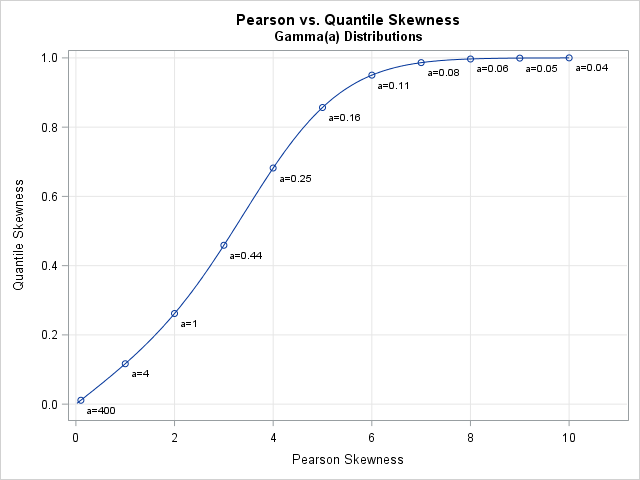The graph shows a nonlinear relationship between the two skewness measures. This graph is for the Gamma distribution; other distributions would have a different shape. If a distribution has a parameter value for which the distribution is symmetric, then the graph will go through the point (0,0). For highly skewed distributions, the quantile skewness will approach ±1 as the Pearson skewness approaches ±∞.

### Alternative quantile definitions

Several researchers have noted that there is nothing special about using the first and third quartiles to measure skewness. An alternative formula (sometimes called Kelly's coefficient of skewness) is to use deciles: γKelly = ((P90 - P50) - (P50 - P10)) / (P90 - P10). Hinkley (1975) considered the q_th and (1-q)_th quantiles for arbitrary values of q.

### Conclusions

The quantile definition of skewness is easy to compute. In fact, you can compute the statistic by hand without a calculator for small data sets. Consequently, the quantile definition provides an easy way to quickly estimate the skewness of data. Since the definition uses only quantiles, the quantile skewness is robust to extreme outliers.

At the same time, the Bowley-Galton quantile definition has several disadvantages. It uses only the central 50% of the data to estimate the skewness. Two different data sets that have the same quartile statistics will have the same quantile skewness, regardless of the shape of the tails of the distribution. And, as mentioned previously, the use of the 25th and 75th percentiles are somewhat arbitrary.

Although the Pearson skewness is widely used in the statistical community, it is worth mentioning that the quantile definition is ideal for use with a box-and-whisker plot. The Q1, Q2, and Q2 quartiles are part of every box plot. Therefore you can visually estimate the quantile skewness as the relative difference between the lengths of the upper and lower boxes.

The post A quantile definition for skewness appeared first on The DO Loop.An important problem in machine learning is the "classification problem." In this supervised learning problem, you build a statistical model that predicts a set of categorical outcomes (responses) based on a set of input features (explanatory variables). You do this by training the model on data for which the outcomes are known. For example, researchers might want to predict the outcomes "Lived" or "Died" for patients with a certain disease. They can use data from a clinical trial to build a statistical model that uses demographic and medical measurements to predict the probability of each outcome.SAS software provides several procedures for building parametric classification models, including the LOGISTIC and DISCRIM procedures. SAS also provides various nonparametric models, such as spline effects, additive models, and neural networks.

For each input, the statistical model predicts an outcome. Thus the model divides the input space into disjoint regions for which the first outcome is the most probable, for which the second outcome is the most probable, and so forth. In many textbooks and papers, the classification problem is illustrated by using a two-dimensional graph that shows the prediction regions overlaid with the training data, as shown in the adjacent image which visualizes a binary outcome and a linear boundary between regions. (Click to enlarge.)

1. The polygon method: A parametric model provides a formula for the boundary between regions. You can use the formula to construct polygonal regions.
2. The contour plot method: If there are two outcomes and the model provides probabilities for the first outcome, then the 0.5 contour divides the feature space into disjoint prediction regions.
3. The background grid method: You can evaluate the model on a grid of points and color each point according to the predicted outcome. You can use small markers to produce a faint indication of the prediction regions, or you can use large markers if you want to tile the graph with color.

This article uses logistic regression to discriminate between two outcomes, but the principles apply to other methods as well. The SAS documentation for the DISCRIM procedure contains some macros that visualize the prediction regions for the output from PROC DISCRIM.

### A logistic model to discriminate two outcomes

To illustrate the classification problem, consider some simulated data in which the Y variable is a binary outcome and the X1 and X2 variable are continuous explanatory variables. The following call to PROC LOGISTIC fits a logistic model and displays the parameter estimates. The STORE statement creates an item store that enables you to evaluate (score) the model on future observations. The DATA step creates a grid of evenly spaced points in the (x1, x2) coordinates, and the call to PROC PLM scores the model at those locations. In the PRED data set, GX and GY are the coordinates on the regular grid and PREDICTED is the probability that Y=1.

```proc logistic data=LogisticData; model y(Event='1') = x1 x2; store work.LogiModel; /* save model to item store */ run;   data Grid; /* create grid in (x1,x2) coords */ do x1 = 0 to 1 by 0.02; do x2 = -7.5 to 7.5 by 0.3; output; end; end; run;   proc plm restore=work.LogiModel; /* use PROC PLM to score model on a grid */ score data=Grid out=Pred(rename=(x1=gx x2=gy)) / ilink; /* evaluate the model on new data */ run;```

### The polygon methodThis method is only useful for simple parametric models. Recall that the logistic function is 0.5 when its argument is zero, so the level set for 0 of the linear predictor divides the input space into prediction regions. For the parameter estimates shown to the right, the level set {(x1,x2) | 2.3565 -4.7618*x1 + 0.7959*x2 = 0} is the boundary between the two prediction regions. This level set is the graph of the linear function x2 = (-2.3565 + 4.7618*x1)/0.7959. You can compute two polygons that represent the regions: let x1 vary between [0,1] (the horizontal range of the data) and use the formula to evaluate x2, or assign x2 to be the minimum or maximum vertical value of the data.

After you have computed polygonal regions, you can use the POLYGON statement in PROC SGPLOT to visualize the regions. The graph is shown at the top of this article. The drawbacks of this method are that it requires a parametric model for which one variable is an explicit function of the other. However, it creates a beautiful image!

### The contour plot methodGiven an input value, many statistical models produce probabilities for each outcome. If there are only two outcomes, you can plot a contour plot of the probability of the first outcome. The 0.5 contour divides the feature space into disjoint regions.

There are two ways to create such a contour plot. The easiest way is to use the EFFECTPLOT statement, which is supported in many SAS/STAT regression procedures. The following statements show how to use the EFFECTPLOT statement in PROC LOGISTIC to create a contour plot, as shown to the right:

```proc logistic data=LogisticData; model y(Event='1') = x1 x2; effectplot contour(x=x1 y=x2); /* 2. contour plot with scatter plot overlay */ run;```

Unfortunately, not every SAS procedure supports the EFFECTPLOT statement. An alternative is to score the model on a regular grid of points and use the Graph Template Language (GTL) to create a contour plot of the probability surface. You can read my previous article about how to use the GTL to create a contour plot.

The drawback of this method is that it only applies to binary outcomes. The advantage is that it is easy to implement, especially if the modeling procedure supports the EFFECTPLOT statement.

### The background grid method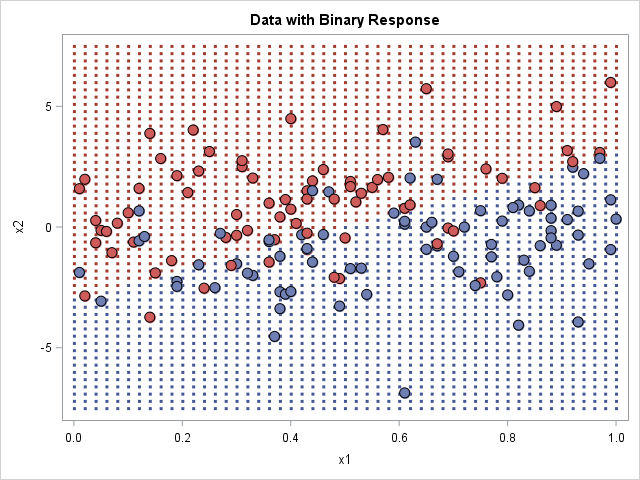In this method, you score the model on a grid of points to obtain the predicted outcome at each grid point. You then create a scatter plot of the grid, where the markers are colored by the outcome, as shown in the graph to the right.

When you create this graph, you get to choose how large to make the dots in the background. The image to the right uses small markers, which is the technique used by Hastie, Tibshirani, and Friedman in their book The Elements of Statistical Learning. If you use square markers and increase the size of the markers, eventually the markers tile the entire background, which makes it look like the polygon plot at the beginning of this article. You might need to adjust the vertical and horizontal pixels of the graph to get the background markers to tile without overlapping each other.

This method has several advantages. It is the most general method and can be used for any procedure and for any number of outcome categories. It is easy to implement because it merely uses the model to predict the outcomes on a grid of points. The disadvantage is that choosing the size of the background markers is a matter of trial and error; you might need several attempts before you create a graph that looks good.

### Summary

This article has shown several techniques for visualizing the predicted outcomes for a model that has two independent variables. The first model is limited to simple parametric models, the second is restricted to binary outcomes, and the third is a general technique that requires scoring the model on a regular grid of inputs. Whichever method you choose, PROC SGPLOT and the Graph Template Language in SAS can help you to visualize different methods for the classification problem in machine learning.

The post 3 ways to visualize prediction regions for classification problems appeared first on The DO Loop.A SAS customer asked how to use SAS to conduct a Z test for the equality of two proportions. He was directed to the SAS Usage Note "Testing the equality of two or more proportions from independent samples." The note says to "specify the CHISQ option in the TABLES statement of PROC FREQ to compute this test," and then adds "this is equivalent to the well-known Z test for comparing two independent proportions."

You might wonder why a chi-square test for association is equivalent to a Z test for the equality of proportions. You might also wonder if there is a direct way to test the equality of proportions. This article implements the well-known test for proportions in the DATA step and compares the results to the chi-square test results. It also shows how to get this test directly from PROC FREQ by using the RISKDIFF option.

### A chi-square test for association in SAS

The SAS Usage Note poses the following problem: Suppose you want to compare the proportions responding "Yes" to a question in independent samples of 100 men and 100 women. The number of men responding "Yes" is observed to be 30 and the number of women responding Yes was 45.

You can create the data by using the following DATA step, then call PROC FREQ to analyze the association between the response variable and gender.

```data Prop; length Group \$12 Response \$3; input Group Response N; datalines; Men Yes 30 Men No 70 Women Yes 45 Women No 55 ;   proc freq data=Prop order=data; weight N; tables Group*Response / chisq; run;```As explained in the PROC FREQ documentation, the Pearson chi-square statistic indicates an association between the variables in the 2 x 2 table. The results show that the chi-square statistic (for 1 degree of freedom) is 4.8, which corresponds to a p-value of 0.0285. The test indicates that we should reject the null hypothesis of no association at the 0.05 significance level.

As stated in the SAS Usage Note, this association test is equivalent to a Z test for whether the proportion of males who responded "Yes" equals the proportion of females who responded "Yes." The equivalence relies on a fact from probability theory: a chi-square random variable with 1 degree of freedom is the square of a random variable from the standard normal distribution. Thus the square root of the chi-square statistic is the Z statistic (up to a sign) that you get from the test of equality of two proportion. Therefore the Z statistic should be z = ±sqrt(4.8) = ±2.19. The p-value is unchanged.

### Z test for the equality of two proportions: A DATA step implmentation

For comparison, you can implement the classical Z test by applying the formulas from a textbook or from the course material from Penn State, which includes a section about comparing two proportions. The following DATA step implements the Z test for equality of proportions:

```/* Implement the Z test for pre-summarized statistics. Specify the group proportions and sizes. For formulas, see https://onlinecourses.science.psu.edu/stat414/node/268 */ %let alpha = 0.05; %let N1 = 100; /* total trials in Group1 */ %let Event1= 30; /* Number of events in Group1 */ %let N2 = 100; /* total trials in Group2 */ %let Event2= 45; /* Number of events in Group2 */   %let Side = 2; /* use L, U, or 2 for lower, upper, or two-sided test */ title "Test of H0: p1=p2 vs Ha: p1^=p2"; /* change for Side=L or U */   data zTestProp; p1Hat = &Event1 / &N1; /* observed proportion in Group1 */ var1 = p1Hat*(1-p1Hat) / &N1; /* variance in Group1 */ p2Hat = &Event2 / &N2; /* observed proportion in Group2 */ var2 = p2Hat*(1-p2Hat) / &N2; /* variance in Group2 */ /* use pooled estimate of p for test */ Diff = p1Hat - p2Hat; /* estimate of p1 = p2 */ pHat = (&Event1 + &Event2) / (&N1 + &N2); pVar = pHat*(1-pHat)*(1/&N1 + 1/&N2); /* pooled variance */ SE = sqrt(pVar); /* estimate of standard error */ Z = Diff / SE;   Side = "&Side"; if Side="L" then /* one-sided, lower tail */ pValue = cdf("normal", z); else if Side="U" then /* one-sided, upper tail */ pValue = sdf("normal", Z); /* SDF = 1 - CDF */ else if Side="2" then pValue = 2*(1-cdf("normal", abs(Z))); /* two-sided */ format pValue PVALUE6.4 Z 7.4; label pValue="Pr < Z"; drop var1 var2 pHat pVar; run;   proc print data=zTestProp label noobs; run;```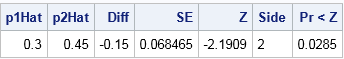The DATA step obtains a test statistic of Z = –2.19, which is one of the square roots of the chi-square statistic in the PROC FREQ output. Notice also that the p-value from the DATA step matches the p-value from the PROC FREQ output.

### Test equality of proportions by using PROC FREQ

There is actually a direct way to test for the equality of two independent proportions: use the RISKDIFF option in the TABLES statement in PROC FREQ. In the documentation, binomial proportions are called "risks," so a "risk difference" is a difference in proportions. (Also, a "relative risk" (the RELRISK option) measures the ratio of two proportions.) Equality of proportions is equivalent to testing whether the difference of proportions (risks) is zero.

As shown in the documentation, PROC FREQ supports many options for comparing proprtions. You can use the following suboptions to reproduce the classical equality of proportions test:

1. EQUAL requests an equality test for the difference in proportion. By default, the Wald interval (METHOD=WALD) is used, but you can choose other intervals.
2. VAR=NULL specifies how to estimate the variance for the Wald interval.
3. (optional) CL=WALD outputs the Wald confidence interval for the difference.

Combining these options gives the following direct computation of the difference between two proportions:

```proc freq data=Prop order=data; weight N; tables Group*Response / riskdiff(equal var=null cl=wald); /* Wald test for equality */ run;```The 95% (Wald) confidence interval is shown in the first table. The confidence interval is centered on the point estimate of the difference (-0.15). The interval does not contain 0, so the difference is significantly different from 0 at the 0.05 significance level.

The second table show the result of the Wald equality test. The "ASE (H0)" row gives the estimate for the (asymptotic) standard error, assuming the null hypothesis. The Z score and the two-sided p-value match the values from the DATA step computation, and the interpretation is the same.

### Summary

In summary, the SAS Usage Note correctly states that the chi-square test of association is equivalent to the Z test for the equality of proportion. To run the Z test explicitly, this article uses the SAS DATA step to implement the test when you have summary statistics. As promised, the Z statistic is one of the square roots of the chi-square statistic and the p-values are the same. The DATA step removes some of the mystery regarding the equivalence between these two tests.

However, writing DATA step code cannot match the convenience of a procedure. For raw or pre-summarized data, you can use the RISKDIFF option in PROC FREQ to run the same test (recast as a difference of proportions or "risks"). To get exactly the same confidence intervals and statistics as the classical test (which is called the Wald test), you need to add a few suboptions. The resulting output matches the DATA step computations.

The post Test for the equality of two proportions in SAS appeared first on The DO Loop.Suppose you roll six identical six-sided dice. Chance are that you will see at least one repeated number. The probability that you will see six unique numbers is very small: only 6! / 6^6 ≈ 0.015.

This example can be generalized. If you draw a random sample with replacement from a set of n items, duplicate values occur much more frequently than most people think. This is the source of the famous "birthday matching problem" in probability, which shows that the probability of duplicate birthdays among a small group of people is higher than most people would guess. (Among 23 people, the probability of a duplicate birthday is more than 50%.)

This fact has implications for bootstrap resampling. Recall that if a sample has n observations, then a bootstrap sample is obtained by sampling n times with replacement from the data. Since most bootstrap samples contain a duplicate of at least one observation, it is also true that most samples omit at least one observation. That raises the question: On average, how many of the original observations are not present in an average bootstrap sample?

### The average bootstrap sample

I'll give you the answer: an average bootstrap sample contains 63.2% of the original observations and omits 36.8%. The book by Chernick and LaBudde (2011, p. 199) states the following result about bootstrap resamples: "If the sample size is large and we [generate many]  bootstrap samples, we will find that on average, approximately 36.8% of the original observations will be missing from the individual bootstrap samples. Another way to look at this is that for any particular observation, approximately 36.8% of the bootstrap samples will not contain it." (Emphasis added.)

You can use elementary probability to derive this result. Suppose the original data contains n observations. A bootstrap sample is generated by sampling with replacement from the data. The probability that a particular observation is not chosen from a set of n observations is 1 - 1/n, so the probability that the observation is not chosen n times is (1 - 1/n)^n. This is the probability that the observation does not appear in a bootstrap sample.

You might remember from calculus that the limit as n → ∞ of (1 - 1/n)^n is 1/e. Therefore, when n is large, the probability that an observation is not chosen is approximately 1/e ≈ 0.368.

### Simulation: the proportion of observations in bootstrap samples

If you prefer simulation to calculation, you can simulate many bootstrap samples and count the proportion of samples that do not contain observation 1, observation 2, and so forth. The average of those proportions should be close to 1/e.

The theoretical result is only valid for large values of n, but let's start with n=6 so that we can print out intermediate results during the simulation. The following SAS/IML program uses the SAMPLE function to simulate rolling six dice a total of 10 times. Each column of the matrix M contains the result of one trial:

```options linesize=128; proc iml; call randseed(54321); n = 6; NumSamples = 10; x = T(1:n); /* the original sample {1,2,...,n} */ M = sample(x, NumSamples//n); /* each column is a draw with replacement */```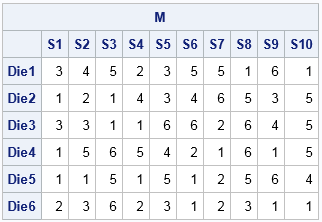The table shows that each sample (column) contains duplicates. The first column does not contain the values {4,5,6}. The second column does not contain the value 6.

Given these samples, what proportion of samples does not contain 1? What proportion does not contain 2, and so forth? For this small simulation, we can answer these questions by visual inspection. The number 1 does not appear in the sample S5, so it does not appear in 0.1 of the samples. The number 2 does not appears in S3, S5, S8, S9, or S10, so it does not appear in 0.5 of the samples. The following SAS/IML statements count the proportion of columns that do not contain each number and then takes the average of those proportions:

```/* how many samples do not contain x, x, etc */ cnt = j(n, 1, .); /* allocate space for results */ do i = 1 to n; Y = (M=x[i]); /* binary matrix */ s = Y[+, ]; /* count for each sample (column) */ cnt[i] = sum(s=0); /* number of samples that do not contain x[i] */ end; prop = cnt / NumSamples; avg_prop = mean(prop); print avg_prop;```The result says that, on the average, a sample does not contain 0.35 of the data values. Let's increase the sample size and the number of bootstrap samples. Change the parameters to the following and rerun the program:

```n = 75; NumSamples = 10000;```

The new estimate is 0.365, which is close to the theoretical value of 1/e. If you like, you can plot a histogram of the n proportions that make up the average:

```title "Proportion of Samples That Do Not Contain an Observation"; title2 "n=75; NumSamples=10000"; call histogram(prop) label="Proprtion" other="refline "+char(avg_prop)+"/axis=x;";```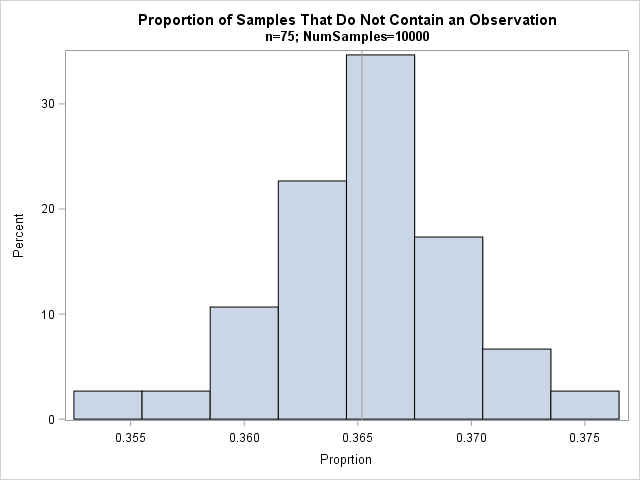Elementary statistical theory tells us that the proportions are approximately normally distributed with mean p=1/e and standard deviation sqrt(p(1-p)/NumSamples) ≈ 0.00482. The mean and standard deviation of the simulated proportions are very close to the theoretical values.

In conclusion, when you draw n items with replacement from a large sample of size n, on average the sample contains 63.2% of the original observations and omits 36.8%. In other words, the average bootstrap sample omits 36.8% of the original data.

The post The average bootstrap sample omits 36.8% of the data appeared first on The DO Loop.In a previous article, I showed two ways to define a log-likelihood function in SAS. This article shows two ways to compute maximum likelihood estimates (MLEs) in SAS: the nonlinear optimization subroutines in SAS/IML and the NLMIXED procedure in SAS/STAT. To illustrate these methods, I will use the same data sets from my previous post. One data set contains binomial data, the other contains data that are lognormally distributed.

### Maximum likelihood estimates for binomial data from SAS/IML

I previously wrote a step-by-step description of how to compute maximum likelihood estimates in SAS/IML. SAS/IML contains many algorithms for nonlinear optimization, including the NLPNRA subroutine, which implements the Newton-Raphson method.

In my previous article I used the LOGPDF function to define the log-likelihood function for the binomial data. The following statements define bounds for the parameter (0 < p < 1) and provides an initial guess of p0=0.5:

```/* Before running program, create Binomial and LN data sets from previous post */   /* Example 1: MLE for binomial data */ /* Method 1: Use SAS/IML optimization routines */ proc iml; /* log-likelihood function for binomial data */ start Binom_LL(p) global(x, NTrials); LL = sum( logpdf("Binomial", x, p, NTrials) ); return( LL ); finish;   NTrials = 10; /* number of trials (fixed) */ use Binomial; read all var "x"; close;   /* set constraint matrix, options, and initial guess for optimization */ con = { 0, /* lower bounds: 0 < p */ 1}; /* upper bounds: p < 1 */ opt = {1, /* find maximum of function */ 2}; /* print some output */ p0 = 0.5; /* initial guess for solution */ call nlpnra(rc, p_MLE, "Binom_LL", p0, opt, con); print p_MLE;```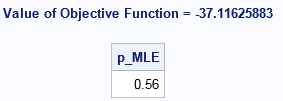The NLPNRA subroutine computes that the maximum of the log-likelihood function occurs for p=0.56, which agrees with the graph in the previous article. We conclude that the parameter p=0.56 (with NTrials=10) is "most likely" to be the binomial distribution parameter that generated the data.

### Maximum likelihood estimates for binomial data from PROC NLMIXED

If you've never used PROC NLMIXED before, you might wonder why I am using that procedure, since this problem is not a mixed modeling regression. However, you can use the NLMIXED procedure for general maximum likelihood estimation. In fact, I sometimes joke that SAS could have named the procedure "PROC MLE" because it is so useful for solving maximum likelihood problems.

PROC NLMIXED has built-in support for computing maximum likelihood estimates of data that follow the Bernoulli (binary), binomial, Poisson, negative binomial, normal, and gamma distributions. (You can also use PROC GENMOD to fit these distributions; I have shown an example of fitting Poisson data.)

The syntax for PROC NLMIXED is very simple for the Binomial data. You use the PARMS statement to supply an initial guess for the parameter p. On the MODEL statement, you declare that you want to model the X variable as Binom(p) where NTrials=10. Be sure to ALWAYS check the documentation for the correct syntax for a binomial distribution. Some functions like PDF, CDF, and RAND use the p parameter as the first argument: Binom(p, NTrials). Some procedure (like the MCMC and NLMIXED) use the p parameter as the second argument: Binom(NTrials, p).

```/* Method 2: Use PROC NLMIXED solve using built-in modeling syntax */ proc nlmixed data=Binomial; parms p = 0.5; * initial value for parameter; NTrials = 10; model x ~ binomial(NTrials, p); run;```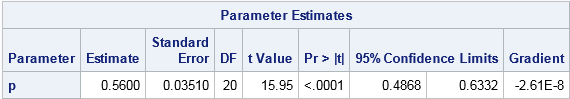Notice that the output from PROC NLMIXED contains the parameter estimate, standard error, and 95% confidence intervals. The parameter estimate is the same value (0.56) as was found by the NLPNRA routine in SAS/IML. The confidence interval confirms what we previously saw in the graph of the log-likelihood function: the function is somewhat flat near the optimum, so a 95% confidence interval is wide: [0.49, 0.63].

### Maximum likelihood estimates for lognormal data

You can use similar syntax to compute MLEs for lognormal data. The SAS/IML syntax is similar to the binomial example, so it is omitted. To view it, download the complete SAS program that computes these maximum likelihood estimates.

PROC NLMIXED does not support the lognormal distribution as a built-in distribution, which means that you need to explicitly write out the log-likelihood function and specify it in the GENERAL function on the MODEL statement. Whereas in SAS/IML you have to use the SUM function to sum the log-likelihood over all observations, the syntax for PROC NLMIXED is simpler. Just as the DATA step has an implicit loop over all observations, the NLMIXED procedure implicitly sums the log-likelihood over all observations. You can use the LOGPDF function, or you can explicitly write the log-density formula for each observation.

If you look up the lognormal distribution in the list of "Standard Definition" in the PROC MCMC documentation, you will see that one parameterization of the lognormal PDF in terms of the log-mean μ and log-standard-deviation σ is
f(x; μ, σ) = (1/(sqrt(2π σ x) exp(-(log(x)-μ)**2 / (2σ**2))
When you take the logarithm of this quantity, you get two terms, or three if you use the rules of logarithms to isolate quantities that do not depend on the parameters:

```proc nlmixed data=LN; parms mu 1 sigma 1; * initial values of parameters; bounds 0 < sigma; * bounds on parameters; sqrt2pi = sqrt(2*constant('pi')); LL = -log(sigma) - log(sqrt2pi*x) /* this term is constant w/r/t (mu, sigma) */ - (log(x)-mu)**2 / (2*sigma**2); /* Alternative: LL = logpdf("Lognormal", x, mu, sigma); */ model x ~ general(LL); run;```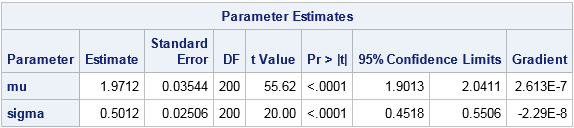The parameter estimates are shown, along with standard errors and 95% confidence intervals. The maximum likelihood estimates for the lognormal data are (μ, σ) = (1.97, 0.50). You will get the same answer if you use the LOGPDF function (inside the comment) instead of the "manual calculation." You will also get the same estimates if you omit the term log(sqrt2pi*x) because that term does not depend on the MLE parameters.

In conclusion, you can use nonlinear optimization in the SAS/IML language to compute MLEs. This approach is especially useful when the computation is part of a larger computational program in SAS/IML. Alternatively, the NLMIXED procedure makes it easy to compute MLEs for discrete and continuous distributions. For some simple distributions, the log-likelihood functions are built into PROC NLMIXED. For others, you can specify the log likelihood yourself and find the maximum likelihood estimates by using the GENERAL function.

The post Two ways to compute maximum likelihood estimates in SAS appeared first on The DO Loop.Maximum likelihood estimation (MLE) is a powerful statistical technique that uses optimization techniques to fit parametric models. The technique finds the parameters that are "most likely" to have produced the observed data. SAS provides many tools for nonlinear optimization, so often the hardest part of maximum likelihood is writing down the log-likelihood function. This article shows two simple ways to construct the log-likelihood function in SAS. For simplicity, this article describes fitting the binomial and lognormal distributions to univariate data.

### Always use the log-likelihood function!

Although the method is known as maximum likelihood estimation, in practice you should optimize the log-likelihood function, which is numerically superior to work with. For an introduction to MLE, including the definitions of the likelihood and log-likelihood functions, see the Penn State Online Statistics Course, which is a wonderful reference.

MLE assumes that the observed data x={x1, x2, ..., xn} are independently drawn from some population. You want to find the most likely parameters θ = (θ1,...,θk) such that the data are fit by the probability density function (PDF), f(x; θ). Since the data are independent, the probability of observing the data is the product Πi f(xi, θ), which is the likelihood function L(θ | x). If you take the logarithm, the product becomes a sum. The log-likelihood function is
LL(θ | x) = Σi log( f(xi, θ) )

This formula is the key. It says that the log-likelihood function is simply the sum of the log-PDF function evaluated at the data values. Always use this formula. Do not ever compute the likelihood function (the product) and then take the log, because the product is prone to numerical errors, including overflow and underflow.

### Two ways to construct the log-likelihood function

There are two simple ways to construct the log-likelihood function in SAS:

### Example: The log-likelihood function for the binomial distribution

A coin was tossed 10 times and the number of heads was recorded. This was repeated 20 times to get a sample. A student wants to fit the binomial model X ~ Binom(p, 10) to estimate the probability p of the coin landing on heads. For this problem, the vector of MLE parameters θ is merely the one parameter p.

Recall that if you are using SAS/IML to optimize an objective function, the parameter that you are trying to optimize should be the only argument to the function, and all other parameters should be specified on the GLOBAL statement. Thus one way to write a SAS/IML function for the binomial log-likelihood function is as follows:

```proc iml; /* Method 1: Use LOGPDF. This method works in DATA step as well */ start Binom_LL1(p) global(x, NTrials); LL = sum( logpdf("Binomial", x, p, NTrials) ); return( LL ); finish;   /* visualize log-likelihood function, which is a function of p */ NTrials = 10; /* number of trials (fixed) */ use Binomial; read all var "x"; close;   p = do(0.01, 0.99, 0.01); /* vector of parameter values */ LL = j(1, ncol(p), .); do i = 1 to ncol(LL); LL[i] = Binom_LL1( p[i] ); /* evaluate LL for a sequence of p */ end;   title "Graph of Log-Likelihood Function"; title2 "Binomial Distribution, NTrials=10"; call series(p, LL) grid={x y} xvalues=do(0,1,0.1) label={"Probability of Sucess (p)", "Log Likelihood"};```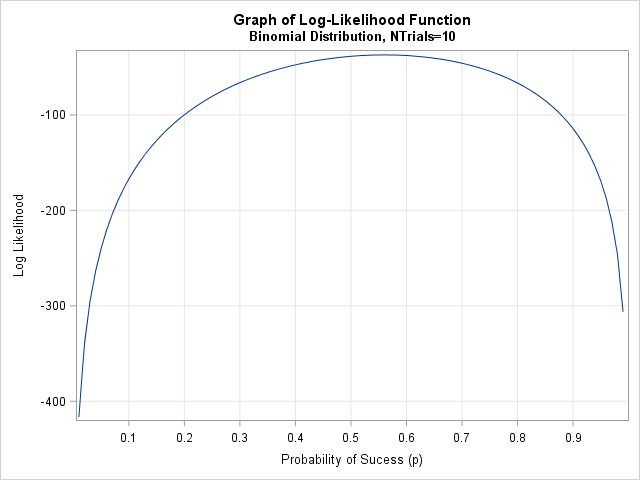Notice that the data is constant and does not change. The log likelihood is considered to be a function of the parameter p. Therefore you can graph the function for representative values of p, as shown. The graph clearly shows that the log likelihood is maximal near p=0.56, which is the maximum likelihood estimate. The graph is fairly flat near its optimal value, which indicates that the estimate has a wide standard error. A 95% confidence interval for the parameter is also wide. If the sample contained 100 observations instead of only 20, the log-likelihood function might have a narrower peak.

Notice also that the LOGPDF function made this computation very easy. You do not need to worry about the actual formula for the binomial density. All you have to do is sum the log-density at the data values.

In contrast, the second method requires a little more work, but can handle any distribution for which you can compute the density function. If you look up the formula for the binomial PDF in the MCMC documentation, you see that
PDF(x; p, NTrials) = comb(NTrials, x) * p**x * (1-p)**(NTrials-x)
where the COMB function computes the binomial coefficient "NTrials choose x." There are three terms in the PDF that are multiplied together. Therefore when you apply the LOG function, you get the sum of three terms. You can use the LCOMB function in SAS to evaluate the logarithm of the binomial coefficients in an efficient manner, as follows:

```/* Method 2: Manually compute log likelihood by using formula */ start Binom_LL2(p) global(x, NTrials); LL = sum(lcomb(NTrials, x)) + log(p)*sum(x) + log(1-p)*sum(NTrials-x); return( LL ); finish;   LL2 = Binom_LL2(p); /* vectorized function, so no need to loop */```

The second formulation has an advantage in a vector language such as SAS/IML because you can write the function so that it can evaluate a vector of values with one call, as shown. It also has the advantage that you can modify the function to eliminate terms that do not depend on the parameter p. For example, if your only goal is maximize the log-likelihood function, you can omit the term sum(lcomb(NTrials, x)) because that term is a constant with respect to p. That reduces the computational burden. Of course, if you omit the term then you are no longer computing the exact binomial log likelihood.

### Example: The log-likelihood function for the lognormal distribution

In a similar way, you can use the LOGPDF or the formula for the PDF to define the log-likelihood function for the lognormal distribution. For brevity, I will only show the SAS/IML functions, but you can download the complete SAS program that defines the log-likelihood function and computes the graph.

The following SAS/IML modules show two ways to define the log-likelihood function for the lognormal distribution. For the lognormal distribution, the vector of parameters θ = (μ, σ) contains two parameters.

```/* Method 1: use LOGPDF */ start LogNormal_LL1(param) global(x); mu = param; sigma = param; LL = sum( logpdf("Lognormal", x, mu, sigma) ); return( LL ); finish;   /* Method 2: Manually compute log likelihood by using formula PDF(x; p, NTrials) = comb(NTrials,x) # p##x # (1-p)##(NTrials-x) */ start LogNormal_LL2(param) global(x); mu = param; sigma = param; twopi = 2*constant('pi'); LL = -nrow(x)/2*log(twopi*sigma##2) - sum( (log(x)-mu)##2 )/(2*sigma##2) - sum(log(x)); /* this term is constant w/r/t (mu, sigma) */ return( LL ); finish;```

The function that uses the LOGPDF function is simple to write. The second method is more complicated because the lognormal PDF is more complicated than the binomial PDF. Nevertheless, the complete log-likelihood function only requires a few SAS/IML statements.

For completeness, the contour plot on this page shows the log-likelihood function for 200 simulated observations from the Lognormal(2, 0.5) distribution. The parameter estimates are (μ, σ) = (1.97, 0.5).### Summary

This article has shown two simple ways to define a log-likelihood function in SAS. You can sum the values of the LOGPDF function evaluated at the observations, or you can manually apply the LOG function to the formula for the PDF function. The log likelihood is regarded as a function of the parameters of the distribution, even though it also depends on the data. For distributions that have one or two parameters, you can graph the log-likelihood function and visually estimate the value of the parameters that maximize the log likelihood.

Of course, SAS enables you to numerically optimize the log-likelihood function, thereby obtaining the maximum likelihood estimates. My next blog post shows two ways to obtain maximum likelihood estimates in SAS.

The post Two simple ways to construct a log-likelihood function in SAS appeared first on The DO Loop.If you toss a coin 28 times, you would not be surprised to see three heads in a row, such as ...THHHTH.... But what about eight heads in a row? Would a sequence such as THHHHHHHHTH... be a rare event?

This question popped into my head last weekend as I attended my son's graduation ceremony. As the students marched in, I noticed that men were dressed in green cap and gowns, whereas the women were dressed in white. They entered in alphabetical order, which randomized the men and women. They filed into 12 rows that each contained 28 seats. Thus each row is like an independent toss of a coin, with green and white representing heads and tails, respectively.When the students entered the ninth row from the left (fourth from the right), I noticed a sequence of eight consecutive "little green men," which is highlighted in red in the picture on this page. (Click to enlarge.) I wish I had a photo of the students seated in their chairs because the effect is more dramatic when the green mortarboards are all aligned. But take my word for it: the long sequence of green was very noticeable.

The picture shows that there was actually a row to the extreme left that was partially filled. For the purpose of this article, ignore the partial row. In the 12 full rows, the number of men in each row is (from left to right) {15, 15, 14, 11, 16, 16, 15, 10, 20, 9, 14, 13}. Remarkably, this adds to 168, so the proportion of men is exactly 0.5 of the 12 x 28 = 336 students.

### Simulate the binary pattern

You can simulate the students by generating 336 random binary values arranged on a 12 x 28 grid. Since this was the graduating class of 2017, I used 2017 as the random number seed in the following DATA step:

```%let NumRows = 12; %let NumInRow= 28; data Graduation; call streaminit(2017); do row = 1 to &NumRows; do seat = 1 to &NumInRow; Male = rand("Bernoulli", 0.5); output; end; end; run;   title "One Simulated Seating Arrangement"; proc sgplot data=Graduation; styleattrs wallcolor=grey DATACONTRASTCOLORS=(white green); scatter x=Row y=Seat / group=male markerattrs=(symbol=SquareFilled); xaxis integer values=(1 to 12); run;```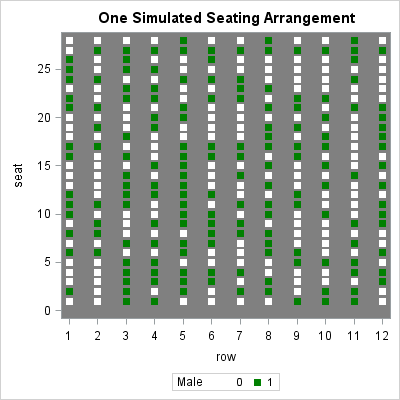If you look at row 5 in the image, you will see a sequence of nine consecutive green markers. The fact that a simulated data set reproduced the graduation scenario on the very first attempt makes me think that this situation is not very rare. However, changing the seed a few times shows that the situation does not always occur.

### Runs in coin tosses

There are 12 rows, each containing 28 students. The event of interest is a row with eight or more consecutive males. The easiest way to compute the probability of this happening is to first compute the probability for one row. Since the rows are assumed to be independent, you can then compute the probability of seeing the event in any of the 12 rows.

A sequence of consecutive events is also called a "run" of events. If you do an internet search for "probability of k heads in a row" or "probability of runs in coin toss", you will find many solutions to this problem. The source I used is a question that was asked on StackExchange about "blocks of events." Whereas many people approach this problem by using a simulation or an explicit recursive mathematical formula, "Neil G" and "COOLSerdash" compute the probability by using a Markov transition matrix, which is easy to create in the SAS/IML matrix language.

The following statements define a function that creates the Markov transition matrix and iterates it to compute the probability that coin will show k consecutive heads in N tosses. The program works for any probability of heads, not merely p=0.5. See the StackExchange article for the explanation:

```proc iml; k = 8; * desired number of correct trials in a row; p = 1/2; * probability of getting a correct trial; N = 28; * Total number of trials;   /* Iterate Markov transition matrix to compute probability of k consecutive heads in N tosses of a coin that has probability p of showing heads */ start ProbConsec(N, p, k); M = j(k+1, k+1, 0); * set up the transition matrix M; M[1, 1:k] = (1-p); * first row, except for last column; M[k+1, k+1] = 1; * lower right corner; do i = 2 to (k+1); M[i, i-1] = p; * subdiagonal elements; end; Mn = M**N; * Calculate M^N; /* Prob that starting in State 1 ends in State (k+1) */ return(Mn[(k+1), 1]); finish;   prob = ProbConsec(N, p, k); print prob;```The result shows that the probability of seeing 8 consecutive heads out of 28 tosses is 0.0426. This is the same probability as observing 8 consecutive men in green in one of the rows at graduation, assuming that alphabetical ordering randomizes men and women. However, remember that there were 12 rows at graduation, so the probability of observing this event in ANY row is higher, as shown below:

```ProbSee0 = (1-prob)##12; * P(Not in Row1 AND ... NOT in Row 12); ProbSeeAny = 1 - ProbSee0; * P(In Row1 OR ... OR in Row 12); print ProbSeeAny ProbSee0;```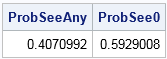The chance of observing exactly eight consecutive men in any of the 12 rows is about 41%. Of course, you can also compute the probability of observing 9, 10, 11, or more consecutive men. When you add up the probabilities, you discover that the cumulative probability of observing an "extreme arrangement" of 8 or more consecutive men is about 0.64. And why stop there? You could extend this analysis to include a sequence of consecutive women!

### Summary

In summary, graduation events can be long, but computing the probabilities of interesting arrangements of the students can help make the time go faster! I wasn't able to compute the probabilities in my head while at the graduation, but it didn't take long to research the problem and solve it with SAS after I got home. I conclude that observing a long sequence of men in a randomized seating arrangement that has 12 rows of 28 seats is not a rare event. In fact, the chance of observing a run of eight or more men is about 64%.

The real lesson for all of us is that we should keep our eyes open and look around. Math and statistics are everywhere!

The post Runs in coin tosses; patterns in random seating appeared first on The DO Loop.According to Hyndman and Fan ("Sample Quantiles in Statistical Packages," TAS, 1996), there are nine definitions of sample quantiles that commonly appear in statistical software packages. Hyndman and Fan identify three definitions that are based on rounding and six methods that are based on linear interpolation. This blog post shows how to use SAS to visualize and compare the nine common definitions of sample quantiles. It also compares the default definitions of sample quantiles in SAS and R.

### Definitions of sample quantiles

Suppose that a sample has N observations that are sorted so that x ≤ x ≤ ... ≤ x[N], and suppose that you are interested in estimating the p_th quantile (0 ≤ p ≤ 1) for the population. Intuitively, the data values near x[j], where j = floor(Np) are reasonable values to use to estimate the quantile. For example, if N=10 and you want to estimate the quantile for p=0.64, then j = floor(Np) = 6, so you can use the sixth ordered value (x) and maybe other nearby values to estimate the quantile.

Hyndman and Fan (henceforth H&F) note that the quantile definitions in statistical software have three properties in common:

• The value p and the sample size N are used to determine two adjacent data values, x[j]and x[j+1]. The quantile estimate will be in the closed interval between those data points. For the previous example, the quantile estimate would be in the closed interval between x and x.
• For many methods, a fractional quantity is used to determine an interpolation parameter, λ. For the previous example, the fraction quantity is (Np - j) = (6.4 - 6) = 0.4. If you use λ = 0.4, then an estimate the 64th percentile would be the value 40% of the way between x and x.
• Each definition has a parameter m, 0 ≤ m ≤ 1, which determines how the method interpolates between adjacent data points. In general, the methods define the index j by using j = floor(Np + m). The previous example used m=0, but other choices include m=0.5 or values of m that depend on p.

Thus a general formula for quantile estimates is q = (1 - λ) x[j]+ λ x[j+1], where λ and j depend on the values of p, N, and a method-specific parameter m.

You can read Hyndman and Fan (1986) for details or see the Wikipedia article about quantiles for a summary. The Wikipedia article points out a practical consideration: for values of p that are very close to 0 or 1, some definitions need to be slightly modified. For example, if p < 1/N, the quantity Np < 1 and so j = floor(Np) equals 0, which is an invalid index. The convention is to return x when p is very small and return x[N] when p is very close to 1.

### Compute all nine sample quantile definitions in SAS

SAS has built-in support for five of the quantile definitions, notably in PROC UNIVARIATE, PROC MEANS, and in the QNTL subroutine in SAS/IML. You can use the QNTLDEF= option to choose from the five definitions. The following table associates the five QNTLDEF= definitions in SAS to the corresponding definitions from H&F, which are also used by R. In R you choose the definition by using the type parameter in the quantile function.It is straightforward to write a SAS/IML function to compute the other four definitions in H&F. In fact, H&F present the quantile interpolation functions as specific instances of one general formula that contains a parameter, which they call m. As mentioned above, you can also define a small value c (which depends on the method) such that the method returns x if p < c, and the method returns x[N] if p ≥ 1 - c.

The following table presents the parameters for computing the four sample quantile definitions that are not natively supported in SAS: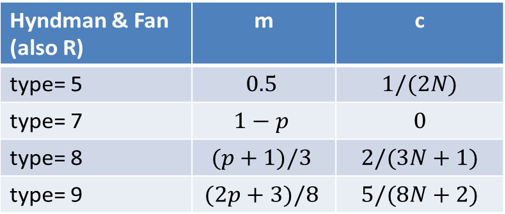### Visualizing the definitions of sample quantilesYou can download the SAS program that shows how to compute sample quantiles and graphs for any of the nine definitions in H&F. The differences between the definitions are most evident for small data sets and when there is a large "gap" between one or more adjacent data values. The following panel of graphs shows the nine sample quantile methods for a data set that has 10 observations, {0 1 1 1 2 2 2 4 5 8}. Each cell in the panel shows the quantiles for p = 0.001, 0.002, ..., 0.999. The bottom of each cell is a fringe plot that shows the six unique data values.

In these graphs, the horizontal axis represents the data and quantiles. For any value of x, the graph estimates the cumulative proportion of the population that is less than or equal to x. Notice that if you turn your head sideways, you can see the quantile function, which is the inverse function that estimates the quantile for each value of the cumulative probability.

You can see that although the nine quantile functions have the same basic shape, the first three methods estimate quantiles by using a discrete rounding scheme, whereas the other methods use a continuous interpolation scheme.

You can use the same data to compare methods. Instead of plotting each quantile definition in its own cell, you can overlay two or more methods. For example, by default, SAS computes sample quantiles by using the type=2 method, whereas R uses type=7 by default. The following graph overlays the sample quantiles to compare the default methods in SAS and R on this tiny data set. The default method in SAS always returns a data value or the average of adjacent data values; the default method in R can return any value in the range of the data.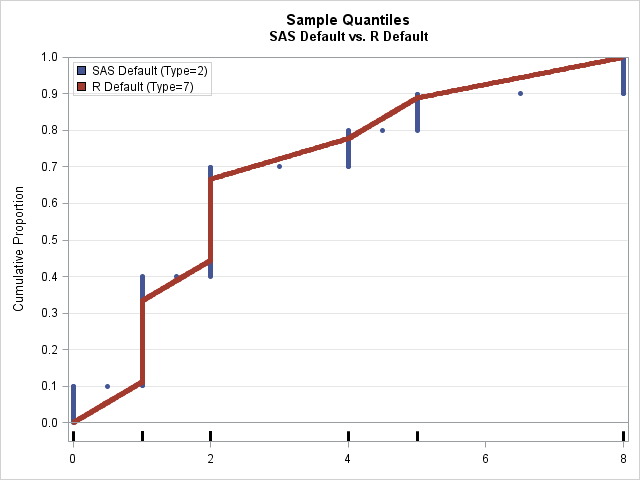### Does the definition of sample quantiles matter?

As shown above, different software packages use different defaults for sample quantiles. Consequently, when you report quantiles for a small data set, it is important to report how the quantiles were computed.

However, in practice analysts don't worry too much about which definition they are using because the difference between methods is typically small for larger data sets (100 or more observations). The biggest differences are often between the discrete methods, which always report a data value or the average between two adjacent data values, and the interpolation methods, which can return any value in the range of the data. Extreme quantiles can also differ between the methods because the tails of the data often have fewer observations and wider gaps.

The following graph shows the sample quantiles for 100 observations that were generated from a random uniform distribution. As before, the two sample quantiles are type=2 (the SAS default) and type=7 (the R default). At this scale, you can barely detect any differences between the estimates. The red dots (type=7) are on top of the corresponding blue dots (type=2), so few blue dots are visible.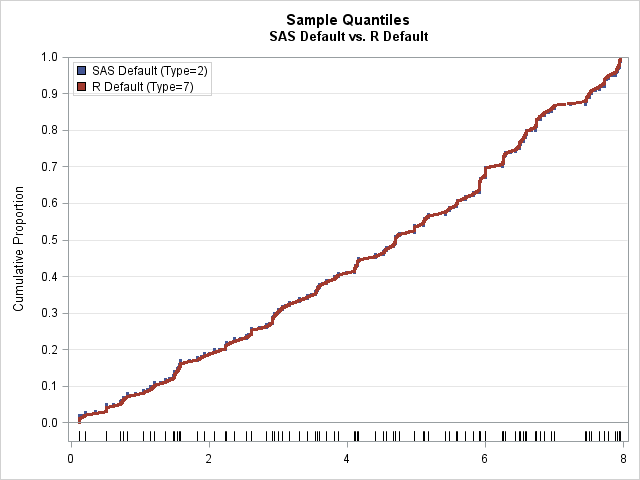So does the definition of the sample quantile matter? Yes and no. Theoretically, the different methods compute different estimates and have different properties. If you want to use an estimator that is unbiased or one that is based on distribution-free computations, feel free to read Hyndman and Fan and choose the definition that suits your needs. The differences are evident for tiny data sets. On the other hand, the previous graph shows that there is little difference between the methods for moderately sized samples and for quantiles that are not near gaps. In practice, most data analysts just accept the default method for whichever software they are using.

In closing, I will mention that there are other quantile estimation methods that are not simple formulas. In SAS, the QUANTREG procedure solves a minimization problem to estimate the quantiles. The QUANTREG procedure enables you to not only estimate quantiles, but also estimate confidence intervals, weighted quantiles, the difference between quantiles, conditional quantiles, and more.

The post Sample quantiles: A comparison of 9 definitions appeared first on The DO Loop.In last week's article about the Flint water crisis, I computed the 90th percentile of a small data set. Although I didn't mention it, the value that I reported is different from the the 90th percentile that is reported in Significance magazine.

That is not unusual. The data only had 20 unique values, and there are many different formulas that you can use to compute sample percentiles (generally called quantiles). Because different software packages use different default formulas for sample quantiles, it is not uncommon for researchers to report different quantiles for small data sets. This article discusses the five percentile definitions that are supported in SAS software.

You might wonder why there are multiple definitions. Recall that a sample quantile is an estimate of a population quantile. Statisticians have proposed many quantile estimators, some of which are based on the empirical cumulative distribution (ECDF) of the sample, which approximates the cumulative distribution function (CDF) for the population. The ECDF is a step function that has a jump discontinuity at each unique data value. Consequently, the inverse ECDF does not exist and the quantiles are not uniquely defined.

### Definitions of sample quantiles

In SAS, you can use the PCTLDEF= option in PROC UNIVARIATE or the QNTLDEF= option in other procedures to control the method used to estimate quantiles. A sample quantile does not have to be an observed data value because you are trying to estimate an unknown population parameter.

For convenience, assume that the sample data are listed in sorted order. In high school, you probably learned that if a sorted sample has an even number of observations, then the median value is the average of the middle observations. The default quantile definition in SAS (QNTLDEF=5) extends this familiar rule to other quantiles. Specifically, if the sample size is N and you ask for the q_th quantile, then when Nq is an integer, the quantile is the data value x[Nq]. However, when Nq is not an integer, then the quantile is defined (somewhat arbitrarily) as the average of the two data x[j] and x[j+1], where j = floor(Nq). For example, if N=10 and you want the q=0.17 quantile, then Nq=1.7, so j=1 and the 17th percentile is reported as the midpoint between the ordered values x and x.

Averaging is not the only choices you can make when Nq is not an integer. The other percentile definitions correspond to making different choices. For example, you could round Nq down (QNTLDEF=3), or you could round it to the nearest integer (QNTLDEF=2). Or you could use linear interpolation (QNTLDEF=1 and QNTLDEF=4) between the data values whose (sorted) indices are closest to Nq. In the example where N=10 and q=0.17, the QNTLDEF=1 interpolated quantile is 0.3 x + 0.7 x.

### Visualizing the definitions for quantiles

The SAS documentation contains the formulas used for the five percentile definitions, but sometimes a visual comparison is easier than slogging through mathematical equations. The differences between the definitions are most apparent on small data sets that contain integer values, so let's create a tiny data set and apply the five definitions to it. The following example has 10 observations and six unique values.

```data Q; input x @@; datalines; 0 1 1 1 2 2 2 4 5 8 ;```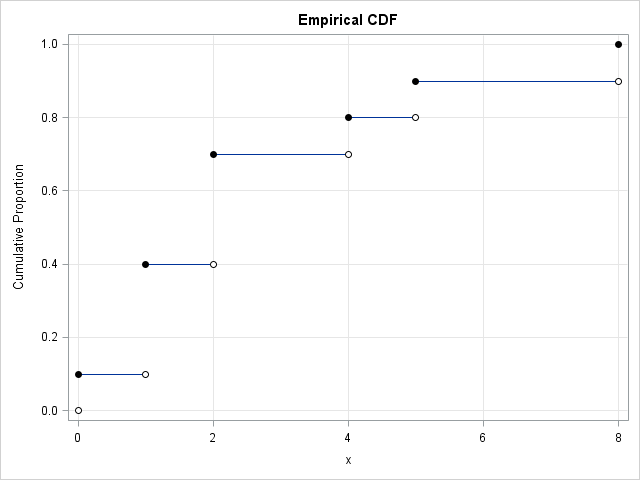You can use PROC UNIVARIATE or other methods to plot the empirical cumulative proportions, as shown. Because the ECDF is a step function, most cumulative proportions values (such as 0.45) are "in a gap." By this I mean that there is no observation t in the data for which the cumulative proportion P(X ≤ t) equals 0.45. Depending on how you define the sample quantiles, the 0.45 quantile might be reported as 1, 1.5, 1.95, or 2.

Since the default definition is QNTLDEF=5, let's visualize the sample quantiles for that definition. You can use the PCTPTS= option on the OUTPUT statement in PROC UNIVARIATE to declare the percentiles that you want to compute. Equivalently, you can use the QNTL function in PROC IML, as below. Regardless, you can ask SAS to find the quantiles for a set of probabilities on a fine grid of points such as {0.001, 0.002, ..., 0.998, 0.999}. You can the graph of the probabilities versus the quantiles to visualize how the percentile definition computes quantiles for the sample data.

```proc iml; use Q; read all var "x"; close; /* read data */ prob = T(1:999) / 1000; /* fine grid of prob values */ call qntl(quantile, x, prob, 5); /* use method=5 definition */ create Pctls var {"quantile" "prob" "x"}; append; close; quit;   title "Sample Percentiles"; title2 "QNTLDEF = 5"; proc sgplot data=Pctls noautolegend; scatter x=quantile y=prob / markerattrs=(size=5 symbol=CircleFilled); fringe x / lineattrs=GraphData2(thickness=3); xaxis display=(nolabel) values=(0 to 8); yaxis offsetmin=0.05 grid values=(0 to 1 by 0.1) label="Cumulative Proportions"; refline 0 1 / axis=y; run;```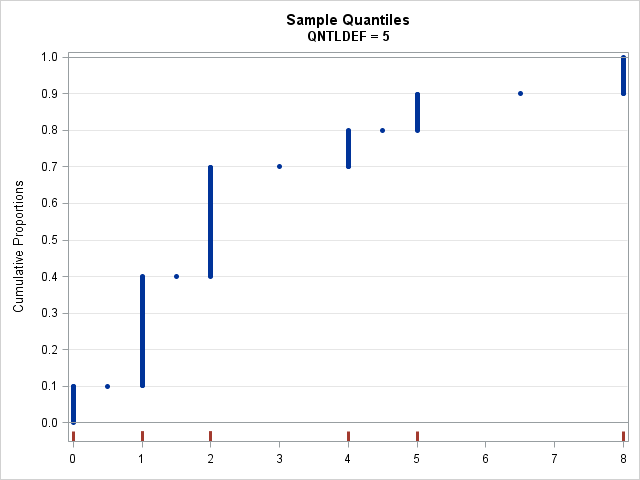For each probability value (Y axis), the graph shows the corresponding sample quantile (X axis) for the default definition in SAS, which is QNTLDEF=5. The X axis also displays red tick marks at the location of the data. You can use this graph to find any quantile. For example, to find the 0.45 quantile, you start at 0.45 on the Y axis, move to the right until you hit a blue marker, and then drop down to the X axis to discover that the 0.45 quantile estimate is 2.

If you prefer to think of the quantiles (the X values) as a function of the probabilities, just interchange the X= and Y= arguments in the SCATTER statement (or turn your head sideways!). Then the quantile function is a step function.

### Comparing all five SAS percentile definitions

It is easy to put a loop around the SAS/IML computation to compute the sample quantiles for the five different definitions that are supported in SAS. The following SAS/IML program writes a data set that contains the sample quantiles. You can use the WHERE statement in PROC PRINT to compare the same quantile across the different definitions. For example, the following displays the 0.45 quantile (45th percentile) for the five definitions:

```/* Compare all SAS methods */ proc iml; use Q; read all var "x"; close; /* read data */ prob = T(1:999) / 1000; /* fine grid of prob values */ create Pctls var {"Qntldef" "quantile" "prob" "x"}; do def = 1 to 5; call qntl(quantile, x, prob, def); /* qntldef=1,2,3,4,5 */ Qntldef = j(nrow(prob), 1, def); /* ID variable */ append; end; close; quit;   proc print data=Pctls noobs; where prob = 0.45; /* compare 0.45 quantile for different definitions */ var Qntldef quantile; run;```You can see that the different definitions lead to different sample quantiles. How do the quantile functions compare? Let's plot them and see:

```ods graphics / antialiasmax=10000; title "Sample Percentiles in SAS"; proc sgpanel data=Pctls noautolegend; panelby Qntldef / onepanel rows=2; scatter x=quantile y=prob/ markerattrs=(size=3 symbol=CircleFilled); fringe x; rowaxis offsetmax=0 offsetmin=0.05 grid values=(0 to 1 by 0.1) label="Cumulative Proportion"; refline 0 1 / axis=y; colaxis display=(nolabel); run;```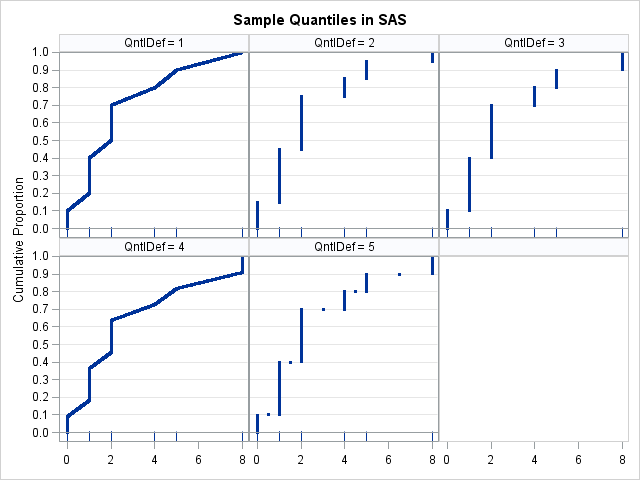The graphs (click to enlarge) show that QNTLDEF=1 and QNTLDEF=4 are piecewise-linear interpolation methods, whereas QNTLDEF=2, 3, and 5 are discrete rounding methods. The default method (QNTLDEF=5) is similar to QNTLDEF=2 except for certain averaged values. For the discrete definitions, SAS returns either a data value or the average of adjacent data values. The interpolation methods do not have that property: the methods will return quantile values that can be any value between observed data values.

If you have a small data set, as in this blog post, it is easy to see how the percentile definitions are different. For larger data sets (say, 100 or more unique values), the five quantile functions look quite similar.

The differences between definitions are most apparent when there are large gaps between adjacent data values. For example, the sample data has a large gap between the ninth and tenth observations, which have the values 5 and 8, respectively. If you compute the 0.901 quantile, you will discover that the "round down" method (QNTLDEF=2) gives 5 as the sample quantile, whereas the "round up" method (QNTLDEF=3) gives the value 8. Similarly, the "backward interpolation method" (QNTLDEF=1) gives 5.03, whereas the "forward interpolation method" (QNTLDEF=4) gives 7.733.

In summary, this article shows how the (somewhat obscure) QNTLDEF= option results in different quantile estimates. Most people just accept the default definition (QNTLDEF=5), but if you are looking for a method that interpolates between data values, rather than a method that rounds and averages, I recommend QNTLDEF=1, which performs linear interpolations of the ECDF. The differences between the definitions are most apparent for small samples and when there are large gaps between adjacent data values.

### Reference